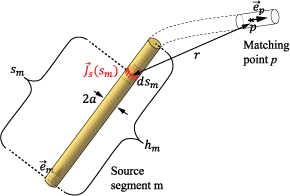## Wire SolverThe following is an abbreviated description of the derivation of the equations solved for by the wire solver. For more detailed information, see .

For PEC (Perfect electrically conducting) wires, the boundary conditions require the tangential component of the total electric field on the wire surface to be zero:

You can also express the equation in terms of the surface current density, ${\stackrel{\to }{J}}_{s}$, on the PEC wire and its spatial derivative. This is done by integrating the effect of the surface current density using a Green's function, $g\left(|\stackrel{\to }{r}-{\stackrel{\to }{r}}^{\text{'}}|\right)$. The Green's function abstractly represents the effect of an infinitesimal surface-current density source located at ${\stackrel{\to }{r}}^{\text{'}}$ on the electric and magnetic fields at an observation point $\stackrel{\to }{r}$. The Green's function for free space propagation is:

where $k=\omega \sqrt{{\epsilon }_{0}{\mu }_{0}}$ is the wave number and ω is the angular frequency. In general, the following steps are needed to solve the electromagnetic (EM) problem and obtain the EM fields in the medium. To represent the EM problem accurately, both longitudinal and transverse components of integrated current are taken into consideration on the wire surface and require that Eq. (1) holds over the entire wire surface. Discretize Eq.(1) over a finite set of points or basis functions to obtain a set of equations. Discretize the surface current-density into a finite set of basis functions to obtain a set of unknowns. Solve the set of equations of unknowns to yield a discrete approximation to the surface currents-densities on the wire. These current densities enables calculation of the electromagnetic fields at any desired point. For thin wires the above steps are exhaustive and, simplify the Green's function to :

also referred to as the thin-wire kernel approximation, where ${r}_{a}=\sqrt{{r}^{2}+{a}^{2}}$ is an approximate average of the distance $|\stackrel{\to }{r}-{\stackrel{\to }{r}}^{\text{'}}|$, r is the distance between the infinitesimal surface current-density source and the wire axis, and a is the wire radius. In addition, the current density on the wire is replaced by the wire current $I=2\pi a|{\stackrel{\to }{J}}_{s}|$|. Using the above approximation, write Eq. (1) as:

where s is the longitudinal location along the wire and $\stackrel{\to }{e}\left(s\right)$ is the unit vector representing the wire orientation at that location. Discretize the wire into a finite number of segments s(m) where m = `1....N`, and then further discretize Eq.(4) into a finite set of equations imposed at points matching p = `1....N` to obtain:

where Im(sm) is the current distribution along the segment m approximated by the polynomial of the form:

with hm being the length of the segment and nm the chosen degree of the polynomial (which is different from segment to segment). Substituting Eq. (6) into (5), the set of equations can be written in a matrix format as:

where [Zp,mi] is the impedance matrix, [Imi] is the vector of unknown coefficients representing the current on the wire, and [Vexp,p] is the excitation vector. More details about the calculation of the elements of [Zp,mi] are given in .

 Popovic,B.C, M.B. Dragovic and A.R. Djordjevic. Analysis and Synthesis of Wire Antennas Research Studies Press, 1982